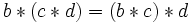# Left-associative elements of loop form subgroup

Jump to: navigation, search

## Statement

Suppose$(L,*)$ is a loop. Then, the left nucleus of$L$, i.e., the set of left-associative elements of$L$, is nonempty and forms a subgroup of$L$. This subgroup is sometimes termed the left kernel of$L$ or the left-associative center of$L$.

## Facts used

1. Left-associative elements of magma form submagma: Further, this submagma is a subsemigroup, and if the whole magma has a neutral element, it has the same neutral element and becomes a monoid.
2. Monoid where every element is right-invertible equals group, which in turn uses equality of left and right inverses in monoid

## Proof

Given: A loop$(L,*)$ with identity element$e$.$S$ is the set of left-associative elements of$L$.

To prove:$S$ is a subgroup of$L$. More explicitly,$(S,*)$ is a group with identity element$e$.

Proof:

Step no. Assertion/construction Facts used Given data used Previous steps used Explanation
1$(S,*)$ is a monoid with identity element$e$. Fact (1)$(L,*)$ has identity element$e$ Follows directly from Fact (1).
2 For any$a \in S$, there is a right inverse of$a$ in$L$, i.e., an element$b \in L$ such that$a * b = e$.$L$ is a loop
3 For any$a \in S$, the right inverse$b$ constructed in Step (2) is in$S$. In other words, for any$c,d \in S$, we have$(b * c) *d = b * (c * d)$.$L$ is a loop, so any equation$a * x = z$ has a unique solution for$x$. Step (2) Using left-associativity of$a$, we get:$a * ((b * c) * d) = (a * (b * c)) * d = ((a * b) * c) * d) = (e * c) * d = c * d$
Similarly,$a * (b * (c * d)) = (a * b) * (c * d) = e * (c * d) = c * d$
Thus, we get:$a * (b * (c * d)) = a * ((b * c) * d) = c * d$.
Since the equation$a * x = c * d$ has a unique solution, we get that$b * (c * d) = (b * c) * d$.
4$(S,*)$ is a monoid with identity element$e$ in which every element has a right inverse. Steps (1), (3) Step-combination direct
5$(S,*)$ is a group with identity element$e$, completing the proof. Fact (2) Step (4) Step-fact combination direct.